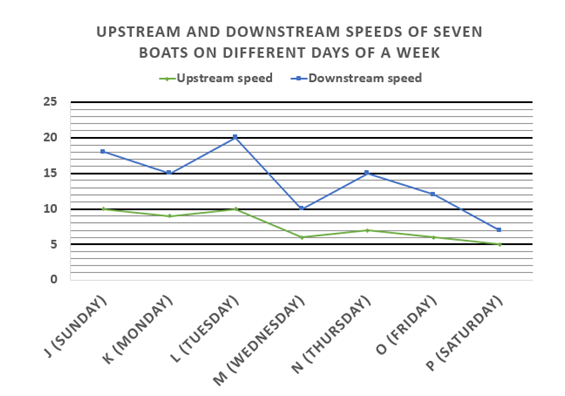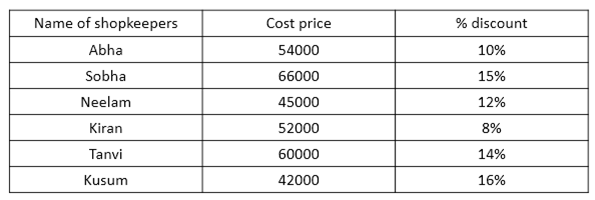# Quantitative Aptitude Questions (Data Interpretation) for SBI PO Mains 2018 Day-155

Dear Readers, SBI is conducting Online Examination for the recruitment of probationary officer. To enrich your preparation here we have providing new series of Data Interpretation – Quantitative Aptitude Questions. Candidates those who are appearing in SBI PO Mains Exams can practice these Quantitative Aptitude average questions daily and make your preparation effective.

Click “Start Quiz” to attend these Questions and view Solutions

Directions (1– 5): Study the following information carefully and answer the questions given below:1) Find the sum of distance travelled by K in 5 hours upstream, O in 8 hours downstream, L in 10 hours downstream and J in 6 hours upstream.

a) 345 Km

b) 329 Km

c) 401 Km

d) 421 Km

e) None of these

2) Sum of the speed of the stream on Sunday and Monday together is what percent of the sum of the speed of the boats in still water on Tuesday and Wednesday together.

a) 30.43%

b) 33.12%

c) 27.68%

d) 23.88%

e) None of these

3) Find the respective ratio of the speed of the stream on Thursday and speed of boat O in still water.

a) 7:5

b) 9:4

c) 5:7

d) 4:9

e) None of these

4) Find the difference between downstream speeds of all the boats together and upstream speed of all the boats together.

a) 44 km/h

b) 34 km/h

c) 38 km/h

d) 40 km/h

e) None of these

5) Speed of boat J in still water is what percent more/less than speed of boat P in still water?

a) 133.33% less

b) 123.33% less

c) 133.33% more

d) 123.33% more

e) None of these

Directions (6 – 10): Study the following information carefully and answer the questions given below:

The following table represents cost price (in Rs.) of a certain article of different shopkeepers and discount allowed by them while selling.6) Sobha marked the price of the article 20% above the cost price and Neelam marked the price of the article 30% above the cost price. Selling price of the article for Sobha is what percent of selling price of the article for Neelam?

a) 112.08%

b) 120.11%

c) 115.89%

d) 130.76%

e) None of these

7) Abha marked the price of the article 15% above the cost price and Kiran marked the price of the article 10% above the cost price. Find the respective ratio of their marked price.

a) 456:533

b) 621:572

c) 533:456

d) 572:621

e) None of these

8) If all the shopkeepers marked the cost prices of the articles by 12%, find the difference between the sum of cost prices of the articles for all the shopkeepers and sum of the marked prices of the articles for all the customers.

a) Rs.32220

b) Rs.34260

c) Rs.31240

d) Rs.38280

e) None of these

9) Selling price of the article for Tanvi is 18% more than the cost price. Find the marked price of the article for Tanvi.

a) Rs.84315.18

b) Rs.82325.58

c) Rs.81324.28

d) Rs.80325.48

e) None of these

10) Cost price of the article for Kusum and Abha together is what percent more/less than the cost price of the article for Sobha and Tanvi together?

a) 21.9% more

b) 23.8% less

c) 21.9 % less

d) 23.8% more

e) None of these

Direction (1-5)

Required sum = 5 x 9 + 8 x 12 + 10 x 20 + 6 x 10

= 45 + 96 + 200 + 60

= 401 Km

Speed of stream on Sunday = (18-10)/2 = 4 kmph

Speed of stream on Monday = (15-9)/2 =3 kmph

Speed of the boat on Tuesday = (20+10)/2 = 15 kmph

Speed of the boat on Wednesday = (10+6)/2 = 8 kmph

Sum of the speeds of the stream on Sunday and Monday together = 4 + 3 = 7 km/h

Sum of the speed of the boats in normal water on Tuesday and Wednesday together

= 15 + 8

= 23 km/h

Required percentage = 7/23 x 100 = 30.43%

Speed of stream on Thursday = (15-7)/2= 4 kmph

Speed of boat O = (12+6)/2= 9 kmph

Required ratio = 4:9

Sum of downstream speed of all the boats = 18 + 15 + 20 + 10 + 15 + 12 + 7

= 97 km/h

Sum of upstream speed of all the boats = 10 + 9 + 10 + 6 + 7 + 6 + 5

= 53 km/h

Required difference = 97 – 53 = 44 kmph

Speed of boat J = (18+10)/2 = 28/2 =1 4 kmph

Speed of boat P = (7+5)/2 = 6 kmph

Required percentage = (14 – 6)/6 x 100

= 8/6 x 100

= 133.33% more

Direction (6-10)

Marked price of the article for Sobha = Rs.79200

Selling price of the article for Sobha = 79200 x 85/100 = Rs.67320

Marked price of the article for Neelam = 45000 x 130/100 = Rs.58500

Selling price of the article for Neelam = 58500 x 88/100 = Rs.51480

Required percentage = 67320/51480 x 100 = 130.76%

Marked price of the article for Abha = 54000 x 115/100 = Rs.62100

Marked price of the article for Kiran = 52000 x 110/100 = Rs.57200

Required ratio = 62100: 57200 = 621:572

Sum of the cost prices of the articles for all the shopkeepers

= 54000 + 66000 + 45000 + 52000 + 60000 + 42000

= Rs.319000

Sum of the marked prices of all the articles for all the shopkeepers

= 319000 x 112/100

= Rs.357280

Required difference = 357280 – 319000 = Rs.38280

We know that

MP x (100 – %d) = cp x (100 + %p)

=>mp x (100 – 14) = 60000 x (100 + 18)

=>mp = 60000 x 118/86

=>mp = Rs.82325.58

Cost price of the article for Kusum and Abha together = 42000 + 54000 = Rs.96000

Cost price of the article for Sobha and Tanvi together = 66000 + 60000 = Rs.126000

Required percentage = (126000 – 96000)/126000 x 100

= 30000/126000 x 100

= 23.8% less

Daily Practice Test Schedule | Good Luck

 Topic Daily Publishing Time Daily News Papers & Editorials 8.00 AM Current Affairs Quiz 9.00 AM Quantitative Aptitude “20-20” 11.00 AM Vocabulary (Based on The Hindu) 12.00 PM General Awareness “20-20” 1.00 PM English Language “20-20” 2.00 PM Reasoning Puzzles & Seating 4.00 PM Daily Current Affairs Updates 5.00 PM Data Interpretation / Application Sums (Topic Wise) 6.00 PM Reasoning Ability “20-20” 7.00 PM English Language (New Pattern Questions) 8.00 PM